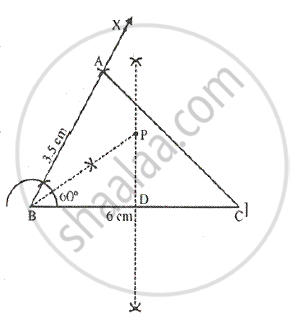# Use Ruler and Compasses Only for this Question. (I) Construct δAbc, Where Ab = 3.5 Cm, Bc = 6cm and ∠Abc = 60°. (Ii) Construct the Locus of Points Inside the Triangle W - Mathematics

Sum

Use ruler and compasses only for this question.
(i) Construct ΔABC, where AB = 3.5 cm, BC = 6cm and ∠ABC = 60°.
(ii) Construct the locus of points inside the triangle which are equidistant from BA and BC.
(iii) Construct the locus of points inside the triangle which are equidistant from B and C.
(iv) Mark the point P which is equidistant from AB, BC and also equidistant from B and C. measure and record the length of PB.

#### Solution

Steps of construction:
i) Draw line BC = 6 cm and an angle CBX = 60°. Cut off AB = 3.5. Join AC, triangle ABC is the required triangle.
ii) Draw perpendicular bisector of BC and bisector of angle B
iii) Bisector of angle B meets bisector of BC at P.
⟹ BP is the required length, where, PB = 3.5 cm
iv) P is the point which is equidistant from BA and BC, also equidistant from B and C.PB = 3.6 cm

Concept: Theorems Based on Loci
Is there an error in this question or solution?

#### APPEARS IN

Selina Concise Maths Class 10 ICSE
Chapter 16 Loci (Locus and Its Constructions)
Exercise 16 (A) | Q 10 | Page 238